Courses

# Test: Acid And Base(Ionic Equilibrium)

## 12 Questions MCQ Test Chemistry for JEE | Test: Acid And Base(Ionic Equilibrium)

Description
This mock test of Test: Acid And Base(Ionic Equilibrium) for JEE helps you for every JEE entrance exam. This contains 12 Multiple Choice Questions for JEE Test: Acid And Base(Ionic Equilibrium) (mcq) to study with solutions a complete question bank. The solved questions answers in this Test: Acid And Base(Ionic Equilibrium) quiz give you a good mix of easy questions and tough questions. JEE students definitely take this Test: Acid And Base(Ionic Equilibrium) exercise for a better result in the exam. You can find other Test: Acid And Base(Ionic Equilibrium) extra questions, long questions & short questions for JEE on EduRev as well by searching above.
QUESTION: 1

### Direction (Q. Nos. 1-15) This section contains 15 multiple choice questions. Each question has four choices (a), (b), (c) and (d), out of which ONLY ONE option is correct. Q. Which of the following is least likely to behave as Lewis base?

Solution:

Electron rich species are called lewis base. Among the given, BF3 is an electron deficient species, so have a capacity of electrons accepting instead of donating that's why it is least likely to act as a lewis base. It is a lewis acid.

QUESTION: 2

### Which of the following is not acid-base conjugate pair?

Solution:

Acid ⇋ Conjugate Base  +  H+
By this definition,
HNO2 ⇋ NO2- + H+
CH3NH3+ ⇋ CH3NH2 + H+
C6H5COOH ⇋ C6H5COO- + H+
H3O+ ⇋ H2O + H+
We can see that the conjugate base of H3O+ is H2O. But it is given that OH- is the C.B. So option d is incorrect.

QUESTION: 3

### Which one of the following molecular hydrides acts as a Lewis acid?

Solution:

B2H6 is an electron deficient species. Soit will act as Lewis acid.

QUESTION: 4

The species present in solution when CO2 dissolved in water, are

[IIT JEE 2006]

Solution:

H2CO3 is formed by dissolution of CO2 into water. This dissociates into H+ and HCO3-. This is the first dissociation of H2CO3. Since HCO3- has another H, it again dissociates to H+ and CO32-. H+ obtained attacks the lone pair of H2O to form H3O+. So H3O+, HCO3-, CO32-, H2CO3 are present in water when CO2 is dissolved in  water.

QUESTION: 5

In the following reaction,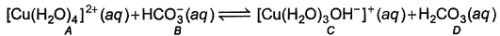Species behaving as Bronsted-Lowry acids are

Solution:

Bronsted lowry acids are those acids which donate H+. In the reaction, A and D are giving H+. So, these both are bronsted lowry acid.

QUESTION: 6

Which of the following will produce a buffer solution when mixed in equal volumes ?

Solution:

For a buffer, we need to have a weak base/acid and its salt. Here, we have a weak base and so we will need its salt. In option c, concentration of strong acid is less than base. So we will have both a weak base and its salt. Therefore, only option c gives us a buffer solution.

QUESTION: 7

PH can be kept constant with help of

Solution:

A buffer is an aqueous solution containing a weak acid and its conjugate base or a weak base and its conjugate acid. It is used to prevent any change in the pH of a solution, regardless of solute. Buffer solutions are used as a means of keeping pH at a nearly constant value in a wide variety of chemical applications.

QUESTION: 8

Acidic strength is in order

Solution:

When we move from left to right, electronegativity of central atom increases. Due to this nature, central atom has more tendency to break its bond with H and acquire negative charge. So, while moving from left to right, acidic strength increase.

QUESTION: 9

Direction Choices for the correct combination of elements from Column I and Column II are given as options (a), (b), (c) and (d), out of which one is correct.

Q. The following thermodynamic quantities are given at 298 K.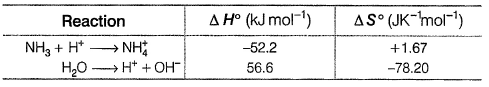Match the parameters given in Column I with their respective values given in Column II.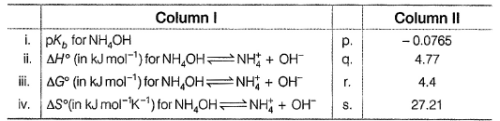Codes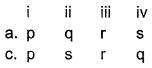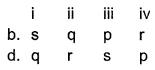Solution:
*Answer can only contain numeric values
QUESTION: 10

What will be the value of pH of 0.01 mol dm–3 CH3COOH (Ka = 1.74 × 10–5)?

Solution: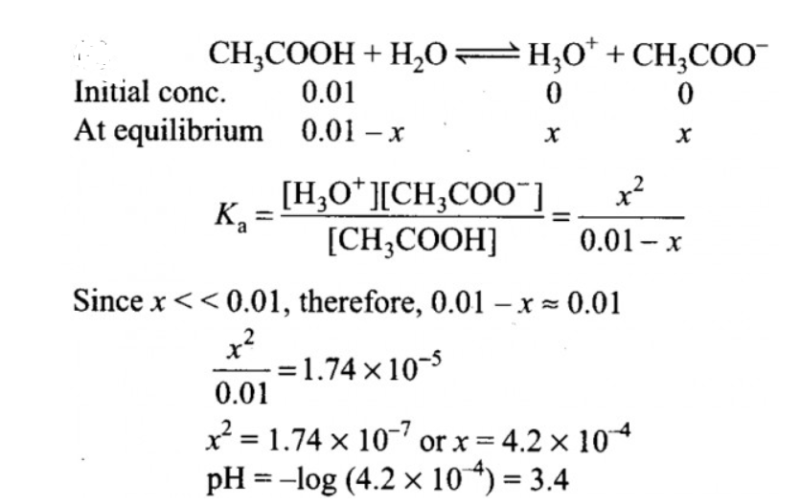*Answer can only contain numeric values
QUESTION: 11

How many of the following are Lewis bases?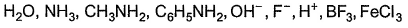Solution:

Total number of compounds acting as Lewis base in the given example is 6. H+ and BF3 are electron deficient so they can't act as Lewis base while FeCl3 acts as Lewis acid so all the other compounds except these three are Lewis base.

*Answer can only contain numeric values
QUESTION: 12

For A- + H2O ⇔ HA + OH-, Kb = 1 x 10-12

Thus, pKa of HA + H2O ⇔ H3O + A- is ........

Solution: